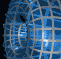Português | English Beyond 3D > A Rotation of Cubes > Mathematics

# The Mathematics of A Rotation of CubesThere are two important mappings involved in this image: rotations and projections. The idea of the shadow is represented mathematically by projection, of which there are two basic types. The first is orthographic projection, which corresponds to a light source that is infinitely far away. In this form of projection, an object's shadow is always the same size as the object itself, regardless of its distance from the shadow. (This form of projection is described in more detail for "Iced Cubes".)

The second form is the stereographic projection. This corresponds to having the light source at a finite distance from the surface on which the shadow falls. In this case, the shadows of objects close to the light source are larger than shadows of objects farther from the light. (Experiment with hand shadows from a lamp in a darkened room to see how this works.) Mathematically, the stereographic projection from the point (0,0,0,d) in four-space onto the xyz hyperplane (i.e., into three-space) is given by the map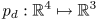where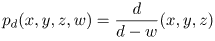for all points where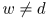.

In our case, the object projected is the hypercube having corners at (±1,±1,±1,±1), and our projection point has d = 4. But our cube is rotated before it is projected. A rotation can be represented by matrix multiplication, for example, to rotate four-space so that the x-axis rotates toward the w-axis through an angle of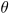, one can use the function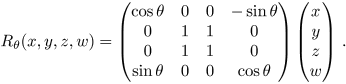Mapping the corners of the hypercube through this rotation map, and then through the projection map gives the positions in three-space of the shadow of the hypercube.

To form the edges of the hypercube, we start by forming the edges of a standard cube in three-space, then add a fourth coordinate that is -1. Then we make a second cube and add a fourth coordinate that is 1. (These two cubes are the ones we colored yellow and orange.) Finally, we add edges between corresponding corners of these two cubes. This forms the skeleton of the hypercube seen in this image.